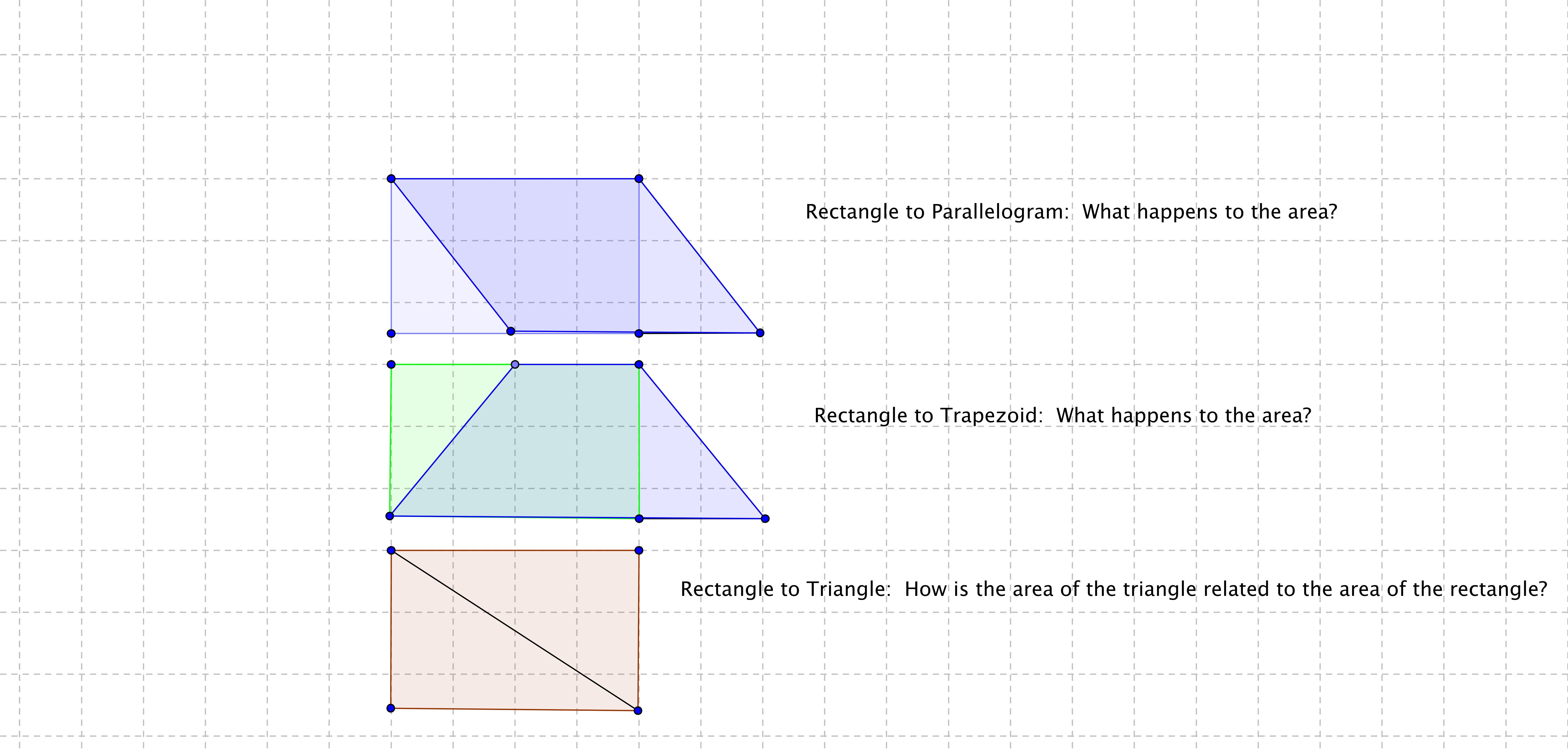# GEOM 1 | Lesson 1 | Try This (Modifying Rectangles) Solutions

Draw a series of shapes starting with a rectangle to show how you can modify the rectangle to create a parallelogram, triangle, and trapezoid, and describe how the area of each is related to each other as the shape changes.In the first figure the area of the rectangle and the area of the parallelogram are the same, note that they have the same base and height.  The area of the triangle is half the area of the rectangle.  The area of the trapezoid is also equal to the area of the rectangle.  In this case the areas of the rectangle, the parallelogram and the trapezoid all have the same area.  The triangles in both the rectangle and the parallelogram would also have the same area.